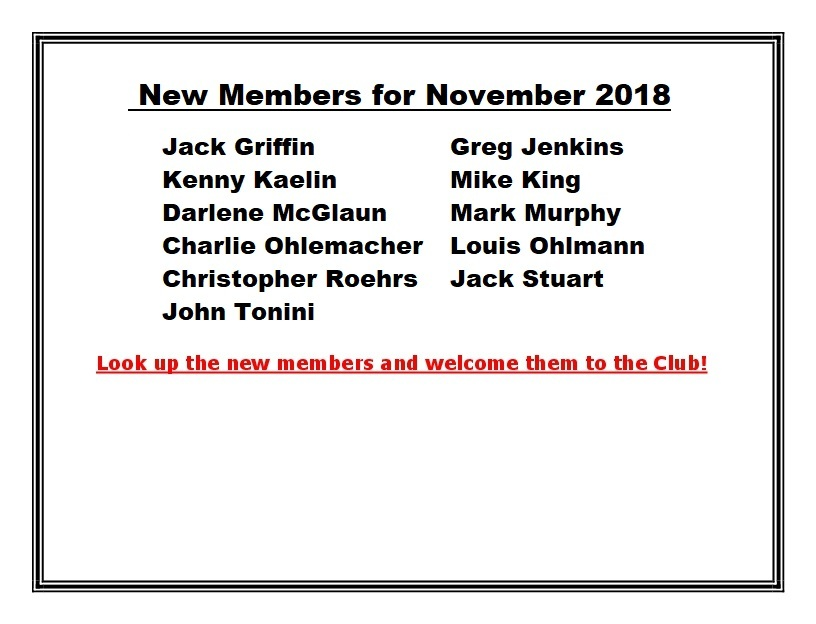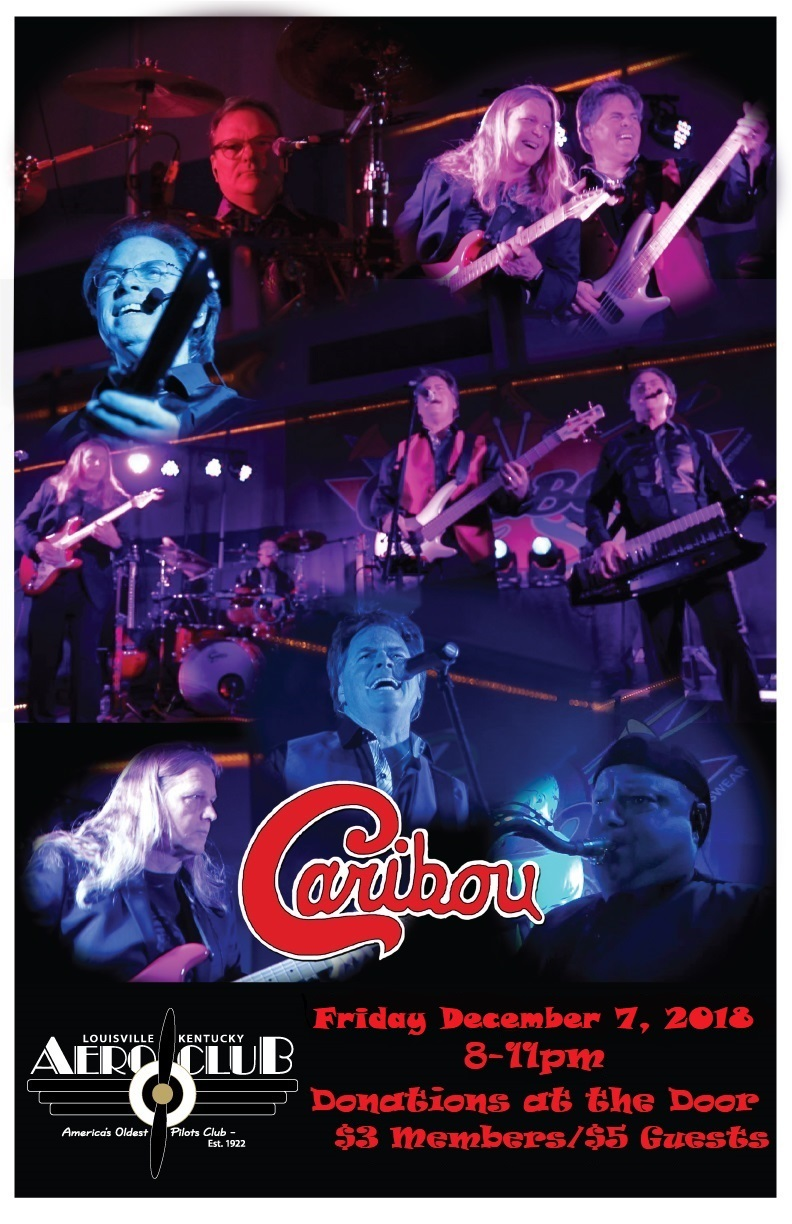Subject: BSU November 28, 2018 From: Aero Club of Louisville Date: 11/28/2018, 8:01 PM
 To: Reg - LaVerne Wilber

BSU November 28, 2018Wednesday November 28, 2018
 table div table+table+table div table{width:100%;padding:0}table div table+table+table div table img{width:96.23%;padding:0;float:none}table div table+table+table div table td{width:100%;padding:0 1.88% 18px}/* styles */The New and Improved Aero Club Web Site is Now Up and Running

Please visit us at the Web Site

www.aeroclub1.com

You will now be able to access the Blue Side Up Newsletter on our website:

www.aeroclub1.com - click on menu item: Blue Side Up - News

 table div table+table+table+table+table div table{width:100%;padding:0}table div table+table+table+table+table div table img{width:96.23%;padding:0;float:none}table div table+table+table+table+table div table td{width:100%;padding:0 1.88% 18px}/* styles */table.module-5{width:38.49%;padding:0}table div table+table+table+table+table+table div table{width:38.49%;float:none;margin-left:auto;margin-right:auto;padding:0}table div table+table+table+table+table+table div table a{border:0 none;text-decoration:none}table div table+table+table+table+table+table div table img{width:100%!important;border:0 none;text-decoration:none}table div table+table+table+table+table+table div table td{width:100%;padding:0}/* styles */
 table div table+table+table+table+table+table+table div table{width:100%;padding:0}table div table+table+table+table+table+table+table div table img{width:96.23%;padding:0;float:none}table div table+table+table+table+table+table+table div table td{width:100%;padding:0 1.88% 18px}/* styles */Please keep your Aero Club Membership information current by sending changes to your address, phone, email, etc. to:

## aeroclub1922@yahoo.com

 table div table+table+table+table+table+table+table+table+table div table{width:100%;padding:0}table div table+table+table+table+table+table+table+table+table div table img{width:96.23%;padding:0;float:none}table div table+table+table+table+table+table+table+table+table div table td{width:100%;padding:0 1.88% 18px}/* styles */table div table+table+table+table+table+table+table+table+table+table div table{width:100%;padding:0}table div table+table+table+table+table+table+table+table+table+table div table img{width:96.23%;padding:0;float:none}table div table+table+table+table+table+table+table+table+table+table div table td{width:100%;padding:0 1.88% 18px}/* styles */table div table+table+table+table+table+table+table+table+table+table+table+table div table{width:100%;padding:0}table div table+table+table+table+table+table+table+table+table+table+table+table div table img{width:96.23%;padding:0;float:none}table div table+table+table+table+table+table+table+table+table+table+table+table div table td{width:100%;padding:0 1.88% 18px}/* styles */table div table+table+table+table+table+table+table+table+table+table+table+table+table+table div table{width:100%;padding:0}table div table+table+table+table+table+table+table+table+table+table+table+table+table+table div table img{width:96.23%;padding:0;float:none}table div table+table+table+table+table+table+table+table+table+table+table+table+table+table div table td{width:100%;padding:0 1.88% 18px}/* styles */table div table+table+table+table+table+table+table+table+table+table+table+table+table+table+table+table div table{width:100%;padding:0}table div table+table+table+table+table+table+table+table+table+table+table+table+table+table+table+table div table img{width:96.23%;padding:0;float:none}table div table+table+table+table+table+table+table+table+table+table+table+table+table+table+table+table div table td{width:100%;padding:0 1.88% 18px}/* styles */table div table+table+table+table+table+table+table+table+table+table+table+table+table+table+table+table+table+table div table{width:100%;padding:0}table div table+table+table+table+table+table+table+table+table+table+table+table+table+table+table+table+table+table div table img{width:96.23%;padding:0;float:none}table div table+table+table+table+table+table+table+table+table+table+table+table+table+table+table+table+table+table div table td{width:100%;padding:0 1.88% 18px}/* styles *//* styles */ Mark your Calendars Friday December 21st, Rob Maupin 8-11pm Friday January 11th, Uptown Band 8-11pm Friday January 18th, The Checkmates 8-11pm Friday February 1st, The Pranksters 8-11pm Sunday February 3rd, Aero Club Super Bowl Party Friday March 1st, Handcar Prophets 8-11pm
 table div table+table+table+table+table+table+table+table+table+table+table+table+table+table+table+table+table+table+table+table div table{width:100%;padding:0}table div table+table+table+table+table+table+table+table+table+table+table+table+table+table+table+table+table+table+table+table div table img{width:96.23%;padding:0;float:none}table div table+table+table+table+table+table+table+table+table+table+table+table+table+table+table+table+table+table+table+table div table td{width:100%;padding:0 1.88% 18px}/* styles *//* styles */ OTHER NOTES NO SMOKING IN THE BUILDING OR ON THE UPPER DECKS. Please help us keep the Club and Club Grounds clean by throwing your trash away and placing cigarette butts in a proper container. Don't just throw them on the ground. Normal Hours of Operation Mon thru Thurs 4-10pm Fri & Sat 4-Midnight Sun 3-10pm